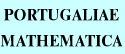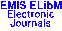PORTUGALIAEMATHEMATICA Vol. 54, No. 2, pp. 215-228 (1997)

Previous Article

Next Article

Contents of this Issue

Other Issues

ELibM Journals

ELibM Home

EMIS Home

## On Para-Kählerian Manifolds $M(J,g)$ and on Skew Symmetric Killing Vector Fields Carried by $M$

### I. Mihai, L. Nicolescu and R. Rosca

Faculty of Mathematics,
Str. Academiei 14, 70109 Bucharest - ROMANIA 59 Avenue Emile Zola,
75015 Paris - FRANCE

Abstract: Para-complex manifolds and, in particular, para-Kählerian manifolds have been for the first time studied by Rashevski [Ra], Libermann [L] and Patterson [Pa]. In the last two decades, several authors have dealt with such type of manifolds, as for instance [R1], [R2], [Cr], [GM], [RMG], [CFG] and some others. A para-Kählerian manifold is a manifold endowed with an almost product structure (called also a para-complex structure) $J$ and a pseudo-Riemannian metric $g$, which satisfy the conditions of compatibility $g\circ(J\times J)=-g$ and $\nabla J=0$, where $\nabla$ is the Levi-Civita connection with respect to $g$.
In the present paper, adopting P. Libermann stand point, we study some properties of a para-Kählerian manifold $M$ and emphasize the case when $M$ carries a non null skew symmetric Killing vector field (in the sense of R. Rosca [R4], [R5]).

Full text of the article: